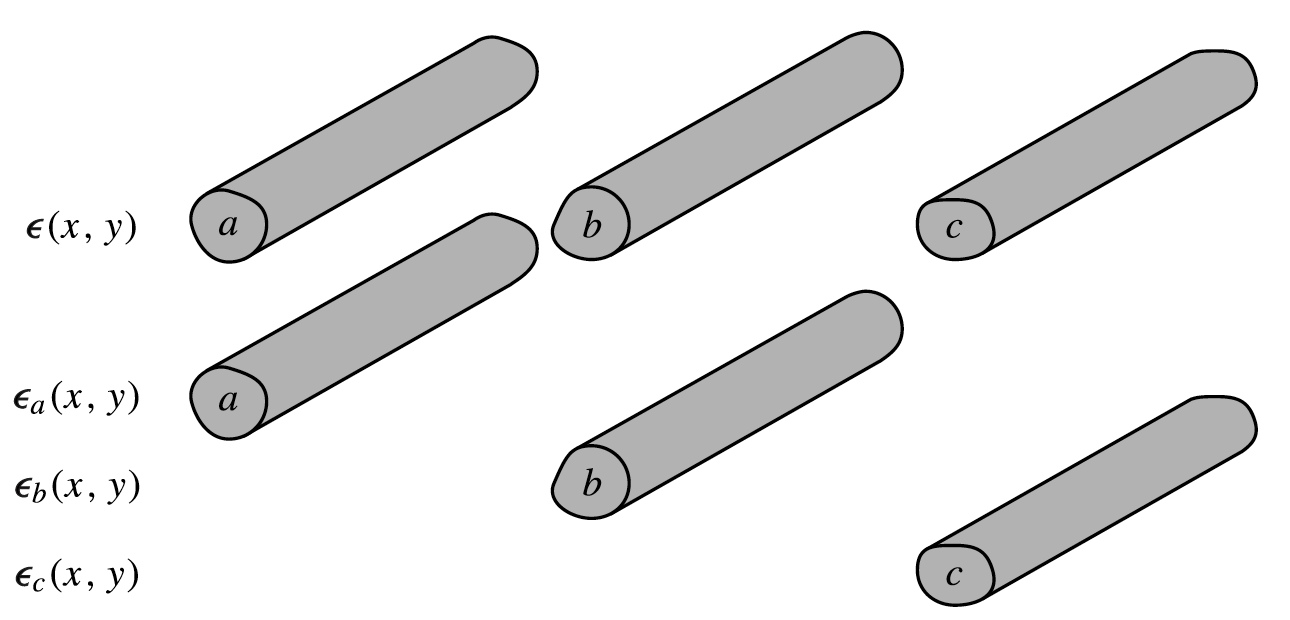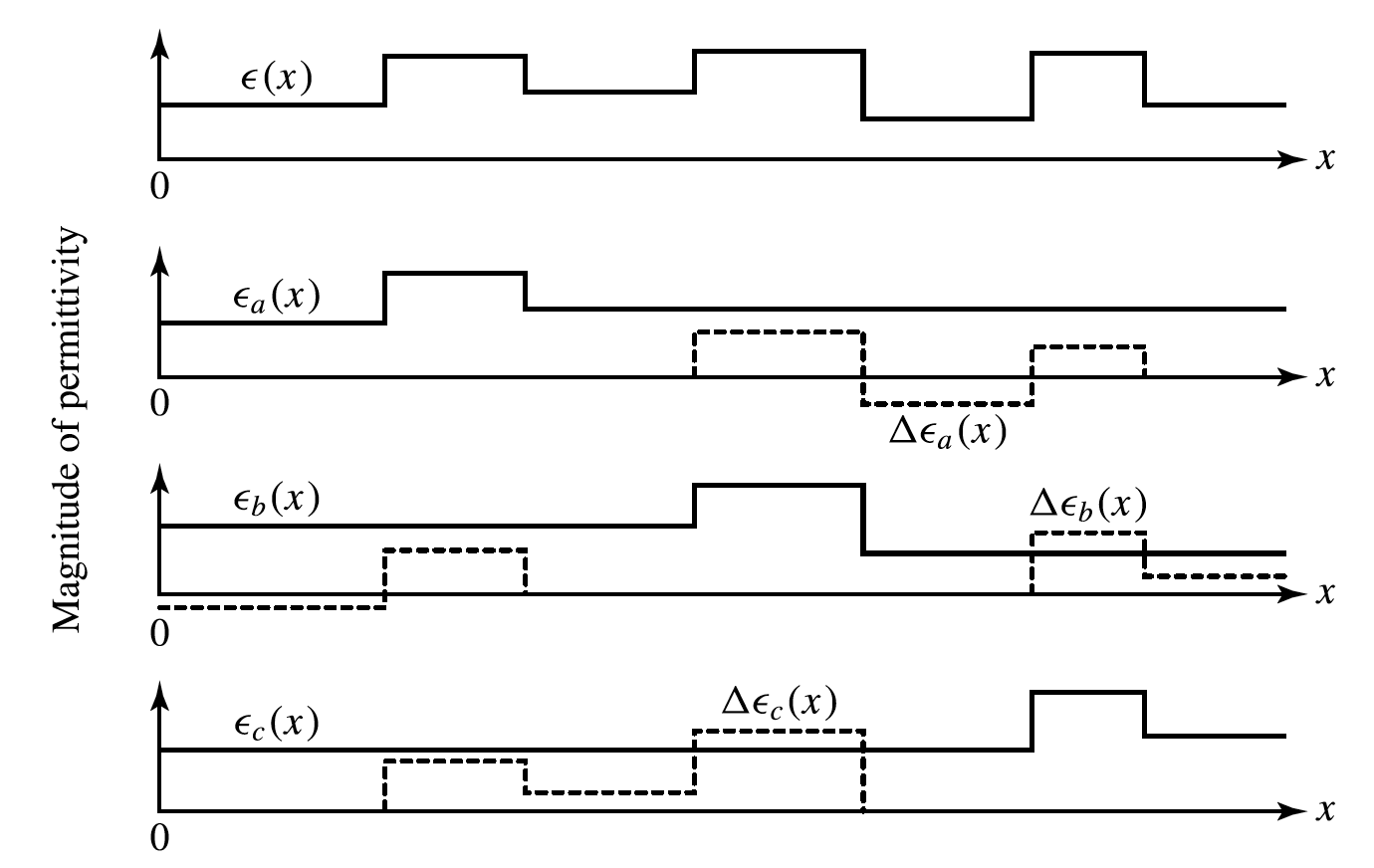# Coupled-Mode Theory

This is a continuation from the previous tutorial - coupled-wave theory.

Coupled-mode theory deals with the coupling of spatial modes of different spatial distributions or different polarizations, or both. Although the theory described in this tutorial is formulated specifically in terms of the coupling of waveguide modes, it can be easily extended to other kind of spatial modes, such as Gaussian spatial modes.

The mode fields in a lossless waveguide can be expressed in the forms of (1) and (2) [refer to the waveguide modes tutorial], which satisfy Maxwell's equations in (8) and (9) [refer to the optical waveguide field equations tutorial]. For fields at a single frequency ω, we can write

$\tag{19}\mathbf{E}_\nu(\mathbf{r})=\boldsymbol{\mathcal{E}}_\nu(x,y)\exp(\text{i}\beta_\nu z)$$\tag{20}\mathbf{H}_\nu(\mathbf{r})=\boldsymbol{\mathcal{H}}_\nu(x,y)\exp(\text{i}\beta_\nu z)$

for the spatial dependence of the mode fields, while the two Maxwell's equations in (8) and (9) become

$\tag{21}\boldsymbol{\nabla}\times\mathbf{E}=\text{i}\omega\mu_0\mathbf{H}$$\tag{22}\boldsymbol{\nabla}\times\mathbf{H}=-\text{i}\omega\epsilon\mathbf{E}$

The normal modes with fields given by (19) and (20) are characteristic solutions of Maxwell's equations in (21) and (22).

Mode expansion

The normal modes are orthogonal and can be normalized to have the orthonormality relation given by (41) [refer to the wave equations tutorial]. They form a basis for linear expansion of any optical field at a given frequency ω in the waveguide:

$\tag{23}\mathbf{E}(\mathbf{r})=\sum_\nu A_\nu\hat{\boldsymbol{\mathcal{E}}}_\nu(x,y)\exp(\text{i}\beta_\nu z)$$\tag{24}\mathbf{H}(\mathbf{r})=\sum_\nu A_\nu\hat{\boldsymbol{\mathcal{H}}}_\nu(x,y)\exp(\text{i}\beta_\nu z)$

where $$\hat{\boldsymbol{\mathcal{E}}}_\nu$$ and $$\hat{\boldsymbol{\mathcal{H}}}_\nu$$ are normalized mode fields satisfying (41) [refer to the wave equations tutorial], and the summation sums over all discrete indices of the guided modes and integrates over all continuous indices of the radiation and evanescent modes.

In an ideal waveguide where these modes are defined, the normal modes do not couple. Then, the expansion coefficients Aν are constants that are independent of x, y, and z.

When there is a spatially dependent perturbation to a waveguide, the modes defined by the unperturbed ideal waveguide are no longer exact normal modes of the perturbed waveguide. They can now be coupled by the perturbation as they propagate along the waveguide. As a result, if the fields are still expanded in terms of then normal modes of the unperturbed waveguide, the expansion coefficients are no longer constants of propagation but vary with z as the fields propagate down the waveguide:

$\tag{25}\mathbf{E}(\mathbf{r})=\sum_\nu A_\nu(z)\hat{\boldsymbol{\mathcal{E}}}_\nu(x,y)\exp(\text{i}\beta_\nu z)$$\tag{26}\mathbf{H}(\mathbf{r})=\sum_\nu A_\nu(z)\hat{\boldsymbol{\mathcal{H}}}_\nu(x,y)\exp(\text{i}\beta_\nu z)$

where again $$\hat{\boldsymbol{\mathcal{E}}}_\nu$$ and $$\hat{\boldsymbol{\mathcal{H}}}_\nu$$ are normalized mode fields, and the summation is taken over all guided, radiation, and evanescent modes.

Single-waveguide mode coupling

We first consider the coupling between normal modes in a single waveguide that is subject to some perturbation. The spatially dependent perturbation to the waveguide can be represented by a perturbing polarization ΔP(r) also at frequency ω. The following Maxwell's equations then replace (21) and (22):

$\tag{27}\boldsymbol{\nabla}\times\mathbf{E}=\text{i}\omega\mu_0\mathbf{H}$$\tag{28}\boldsymbol{\nabla}\times\mathbf{H}=-\text{i}\omega\epsilon\mathbf{E}-\text{i}\omega\Delta\mathbf{P}$

The fields in the perturbed waveguide, which can be expanded as (25) and (26), are governed by these two equations with ΔP ≠ 0. Meanwhile, the normal mode fields of the unperturbed waveguide, which are defined by (21) and (22), also satisfy these two equations with ΔP = 0.

Using (27) and (28), we have

$\tag{29}\boldsymbol{\nabla}\cdot(\mathbf{E}_1\times\mathbf{H}_2^*+\mathbf{E}_2^*\times\mathbf{H}_1)=-\text{i}\omega(\mathbf{E}_1\cdot\Delta\mathbf{P}_2^*-\mathbf{E}_2^*\cdot\Delta\mathbf{P}_1)$

This is the Lorentz reciprocity theorem, which holds for any two arbitrary sets of fields $$(\mathbf{E}_1,\mathbf{H}_1)$$ and $$(\mathbf{E}_2,\mathbf{H}_2)$$.

If we take $$(\mathbf{E}_1,\mathbf{H}_1)$$ to be those of (25) and (26) and $$(\mathbf{E}_2,\mathbf{H}_2)$$ to be the normal mode fields given in (19) and (20), we have ΔP1 = ΔP and ΔP2 = 0. Substituting these into (29) and integrating both sides of the resultant equation over the cross section of the waveguide, we have

\tag{30}\begin{align}\sum_\nu\frac{\text{d}}{\text{d}z}A_\nu(z)\text{e}^{\text{i}(\beta_\nu-\beta_\mu)z}\displaystyle\int\limits_{-\infty}^{\infty}\int\limits_{-\infty}^{\infty}(\hat{\boldsymbol{\mathcal{E}}}_\nu\times\hat{\boldsymbol{\mathcal{H}}}_\mu^*+\hat{\boldsymbol{\mathcal{E}}}_\mu^*\times\hat{\boldsymbol{\mathcal{H}}}_\nu)\cdot\hat{z}\text{d}x\text{d}y\\=\text{i}\omega\text{e}^{-\text{i}\beta_\mu z}\displaystyle\int\limits_{-\infty}^{\infty}\int\limits_{-\infty}^{\infty}\hat{\boldsymbol{\mathcal{E}}}_\mu^*\cdot\Delta\mathbf{P}\text{d}x\text{d}y\end{align}

By applying the orthonormality relation (41) [refer to the wave equations for optical waveguide tutorial], we find from (30) above the following coupled-mode equation:

$\tag{31}\pm\frac{\text{d}A_\nu}{\text{d}z}=\text{i}\omega\text{e}^{-\text{i}\beta_\nu z}\displaystyle\int\limits_{-\infty}^{\infty}\int\limits_{-\infty}^{\infty}\hat{\boldsymbol{\mathcal{E}}}_\nu^*\cdot\Delta\mathbf{P}\text{d}x\text{d}y$

where the plus sign is used when $$\beta_\nu\gt0$$ and mode $$\nu$$ is a forward-propagating mode, and the minus sign is used when $$\beta_\nu\lt0$$ and mode $$\nu$$ is a backward-propagating mode.

The result in (31) can be used for mode coupling caused by any kind of spatially dependent perturbation on the characteristics of the waveguide. For example, $$\Delta\mathbf{P}$$ can be a perturbing polarization due to the effects of nonlinear optical interactions on the fields at frequency $$\omega$$ in the waveguide. For the simple case where the perturbation can be represented by a change in linear polarization as

$\tag{32}\Delta\mathbf{P}=\Delta\epsilon\mathbf{E}=\Delta\epsilon\sum_\nu A_\nu\hat{\boldsymbol{\mathcal{E}}}_\nu\text{e}^{\text{i}\beta_\nu z}$

we have

$\tag{33}\pm\frac{\text{d}A_\nu}{\text{d}z}=\sum_\mu\text{i}\kappa_{\nu\mu}A_\mu\text{e}^{\text{i}(\beta_\mu-\beta_\nu)z}$

where

$\tag{34}\kappa_{\nu\mu}=\omega\displaystyle\int\limits_{-\infty}^{\infty}\int\limits_{-\infty}^{\infty}\Delta\epsilon\hat{\boldsymbol{\mathcal{E}}}_\nu^*\cdot\hat{\boldsymbol{\mathcal{E}}}_\mu\text{d}x\text{d}y$

is the coupling coefficient between mode $$\nu$$ and mode $$\mu$$. This result can also be extended to anisotropic waveguides by simply considering $$\Delta\mathbf{P}$$ to be a polarization involving anisotropy as

$\tag{35}\Delta\mathbf{P}=\Delta\boldsymbol{\epsilon}\cdot\mathbf{E}$

where $$\Delta\boldsymbol{\epsilon}$$ is a tensor. In this situation, the coupled-mode equation is still given by (33), but the coupling coefficient is given by

$\tag{36}\kappa_{\nu\mu}=\omega\displaystyle\int\limits_{-\infty}^{\infty}\int\limits_{-\infty}^{\infty}\hat{\boldsymbol{\mathcal{E}}}_\nu^*\cdot\Delta\boldsymbol{\epsilon}\cdot\hat{\boldsymbol{\mathcal{E}}}_\mu\text{d}x\text{d}y$

In a lossless waveguide, the dielectric tensor is a Hermitian matrix, as discussed in the propagation in an anisotropic medium tutorial. Therefore, $$\Delta\epsilon_{\text{ij}}=\Delta\epsilon_{\text{ji}}^*$$ and

$\tag{37}\kappa_{\nu\mu}=\kappa_{\mu\nu}^*$

in a lossless waveguide.

Multiple-waveguide mode coupling

In an optical structure that consists of more than one waveguide, we can certainly solve Maxwell's equations directly with the boundary conditions defined by the entire structure to find its normal modes. Alternatively, we can divide the structure into separate individual waveguides, expand the fields in terms of the normal modes of the individual waveguides, and treat the problem with a coupled-mode approach.

The first approach can yield exact solutions and is sometimes desirable. However, it is not generally possible to obtain the exact solutions for complicated structures. The coupled-mode approach yields approximate solutions, but it can be applied to most structures without difficulty. In addition, it gives an intuitive picture of how optical waves interact in a multiple-waveguide structure. In the following, we consider the coupled-mode formulation for multiple parallel waveguides.

The concept of dividing a multiple-waveguide structure into a combination of individual single waveguides is illustrated in figure 1 below.Figure 1  Representation of a multiple-waveguide structure in terms of a combination of individual single waveguides

The multiple-waveguide structure is described by $$\boldsymbol{\epsilon}(x,y)$$ while the individual waveguides are described by $$\boldsymbol{\epsilon}_a(x,y)$$, $$\boldsymbol{\epsilon}_b(x,y)$$, $$\boldsymbol{\epsilon}_c(x,y)$$, and so on. The normal modes are solved for each individual waveguide. The fields in the entire structure can be expanded in terms of these normal modes in the same form as that of (25) and (26) but with the index $$\nu$$ representing modes of different individual waveguides. From the standpoint of any individual waveguide $$\nu$$, the entire structure looks like $$\boldsymbol{\epsilon}_\nu(x,y)$$ plus a perturbation of

$\tag{38}\Delta\boldsymbol{\epsilon}_\nu(x,y)=\boldsymbol{\epsilon}(x,y)-\boldsymbol{\epsilon}_\nu(x,y)$

This concept is schematically illustrated in figure 2 below.Figure 2. Schematic diagram of three coupled waveguides showing the decomposition into individual waveguides, in solid curves, plus the corresponding perturbation, in dashed curves, for each of them.

The coupled-mode equation for the multiple-waveguide structure can be obtained by using the reciprocity theorem of (29) and then following a procedure similar to that taken above to obtain the coupled-mode equation for the single waveguide. Because the mathematics is quite involved, we only give the results in the following without detailed derivation.

The coupled-mode equation for a multiple-waveguide structure can still be written in the same form as that of (33):

$\tag{39}\pm\frac{\text{d}A_\nu}{\text{d}z}=\sum_\mu\text{i}\kappa_{\nu\mu}A_\mu\text{e}^{\text{i}(\beta_\mu-\beta_\nu)z}$

where the plus sign is taken if mode $$\nu$$ is forward propagating, and the minus sign is used if it is backward propagating. It is noted that the summation over the index $$\mu$$ runs through the modes of every individual waveguide, not just the modes of one single waveguide. In addition, the coupling coefficients $$\kappa_{\nu\mu}$$ have a complicated form and are best expressed in terms of the matrix elements:

$\tag{40}\kappa_{\nu\mu}=c_{\nu\nu}[\mathbf{c}^{-1}\cdot\tilde{\boldsymbol{\kappa}}]_{\nu\mu}$

where $$c_{\nu\nu}=1$$ if mode $$\nu$$ is forward propagating and $$c_{\nu\nu}=-1$$ if it is backward propagating, as can be seen from (41) below. The elements of the matrices $$\mathbf{c}=[c_{\nu\mu}]$$ and $$\tilde{\boldsymbol{\kappa}}=[\tilde{\kappa}_{\nu\mu}]$$ are given by

$\tag{41}c_{\nu\mu}=\displaystyle\int\limits_{-\infty}^{\infty}\int\limits_{-\infty}^{\infty}(\hat{\boldsymbol{\mathcal{E}}}_\nu^*\times\hat{\boldsymbol{\mathcal{H}}}_\mu+\hat{\boldsymbol{\mathcal{E}}}_\mu\times\hat{\boldsymbol{\mathcal{H}}}_\nu^*)\cdot\hat{z}\text{d}x\text{d}y=c_{\mu\nu}^*$

and

$\tag{42}\tilde{\kappa}_{\nu\mu}=\omega\displaystyle\int\limits_{-\infty}^{\infty}\int\limits_{-\infty}^{\infty}\hat{\boldsymbol{\mathcal{E}}}_\nu^*\cdot\Delta\boldsymbol{\epsilon}_\mu\cdot\hat{\boldsymbol{\mathcal{E}}}_\mu\text{d}x\text{d}y$

respectively.

The coefficient $$c_{\nu\mu}$$ represents the overlap coefficient of $$(\hat{\boldsymbol{\mathcal{E}}}_\nu,\hat{\boldsymbol{\mathcal{H}}}_\nu)$$ and $$(\hat{\boldsymbol{\mathcal{E}}}_\mu,\hat{\boldsymbol{\mathcal{H}}}_\mu)$$, which are the mode fields of different individual waveguides. Note that $$c_{\nu\mu}\ne0$$ in general because modes of different waveguides are not necessarily orthogonal to each other. Because the mode fields used in (41) are normalized, we have $$c_{\nu\nu}=1$$ or $$-1$$, depending on whether the mode $$\nu$$ is forward or backward propagating as mentioned above, and $$|c_{\nu\mu}|\leq1$$.

Note also the difference between the form of $$\tilde{\kappa}_{\nu\mu}$$ and that of the coupling coefficients of modes in a single waveguide given by (36).

In general,

$\tag{43}\tilde{\kappa}_{\nu\mu}\ne\tilde{\kappa}_{\mu\nu}^*\qquad\text{and}\qquad\kappa_{\nu\mu}\ne\kappa_{\mu\nu}^*$

where $$\nu$$ and $$\mu$$ refer to modes of two different waveguides. Therefore, (37) is not always valid for coupling between waveguides. Indeed, it can be shown by using the reciprocity theorem that

$\tag{44}\tilde{\kappa}_{\nu\mu}-\tilde{\kappa}_{\mu\nu}^*=\frac{c_{\nu\mu}+c_{\mu\nu}^*}{2}(\beta_\nu-\beta_\mu)=c_{\nu\mu}(\beta_\nu-\beta_\mu)$

It can be seen that there is a direct relationship between the coupling coefficients and the propagation constants. This is an important relation. It has the following implications:

1. Coupling between two modes is not symmetric, $$\kappa_{\nu\mu}\ne\kappa_{\mu\nu}^*$$, unless $$\beta_\nu=\beta_\mu$$ or $$c_{\nu\mu}=c_{\mu\nu}^*=0$$. This is because the normal modes in different individual waveguides are not necessarily orthogonal to each ther.
2. Coupling of modes of the same order between two identical waveguides is always symmetric, resulting in $$\tilde{\kappa}_{\nu\mu}=\tilde{\kappa}_{\mu\nu}^*$$ and $$\kappa_{\nu\mu}=\kappa_{\mu\nu}^*$$.
3. The relation in (44) applies to modes in a single waveguide as well. In this situation, $$c_{\nu\mu}=c_{\mu\nu}^*=0$$ if $$\nu\ne\mu$$, but $$\tilde{\kappa}_{\nu\mu}=\kappa_{\nu\mu}$$. Therefore, (37) holds in a single waveguide because the normal modes in the same waveguide are always orthogonal to each other.
4. It is not possible to change the coupling between two modes without simultaneously changing their overlap coefficient or their propagation constants.

The next part continues with the Two-Mode Coupling tutorial.